# Underscore.js | _.range()

_.range() function:

• It is used to print the list of elements from the start given as a parameter to the end also a parameter.
• The start and step parameters are optional.
• The default value of start is 0 and that of step is 1.
• In the list formed the start is inclusive and the stop is exclusive.
• The step parameter can be either positive or negative.

Syntax:

`_.range([start], stop, [step])`

Parameters:
It takes three arguments:

• The start (optional)
• The stop
• The step (optional)

Return value:
The returned value is the list from the start till the end (exclusive).

Examples:

1. Passing only the stop parameter to the _.range() function:
The ._range() function takes the element from the list one by one and do the specified operations on the code. Like here the operation is addition of the elements of the list. After adding all the elements, the reduce function ends. Here the starting value of memo is taken as ‘0’.

 `` `<``html``> ` `  `  `<``head``> ` `    ``<``script` `src` `=  ` `"https://cdnjs.cloudflare.com/ajax/libs/underscore.js/1.9.1/underscore-min.js"` `> ` `    `` ` ` ` `  `  `<``body``> ` `    ``<``script` `type``=``"text/javascript"``> ` `        ``console.log(_.range(7)); ` `    `` ` ` ` `  `  ` `

Output: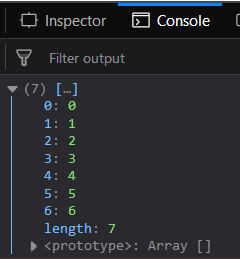2. Passing 2 parameters to the _.range() function:
We can even use this function by passing only 2 parameters, i.e., the start and the stop parameters then also it will have no errors. Like hers the start parameter is 7 which will be included in the list. And the end parameter is 14 which is not included in the list as per the _.range function. So we will take the default parameter of the step parameter which is one. Hence we will get a list from 7 till 13.

 `` `<``html``> ` `  `  `<``head``> ` `    ``<``script` `src` `=  ` `"https://cdnjs.cloudflare.com/ajax/libs/underscore.js/1.9.1/underscore-min.js"` `> ` `    `` ` ` ` `  `  `<``body``> ` `    ``<``script` `type``=``"text/javascript"``> ` `        ``console.log(_.range(7, 14)); ` `    `` ` ` ` `  `  ` `

Output: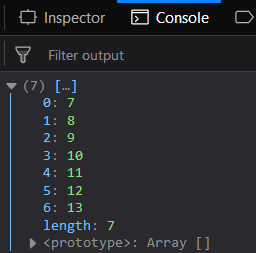3. Passing all the 3 parameters to the _.range() function:
Here we take all the 3 parameters, i.e., the start, stop and the step of the list are mentioned. So, there is no need for the default values. Here the start is from 7 and the step is 3 that means the after 7 the element will be 7+3= 10 in the list. And the calculations will continue in the same manner till the end which is 20 comes.

 `` `<``html``> ` `  `  `<``head``> ` `    ``<``script` `src` `=  ` `"https://cdnjs.cloudflare.com/ajax/libs/underscore.js/1.9.1/underscore-min.js"` `> ` `    `` ` ` ` `  `  `<``body``> ` `    ``<``script` `type``=``"text/javascript"``> ` `        ``console.log(_.range(7, 21, 3)); ` `    `` ` ` ` `  `  ` `

Output: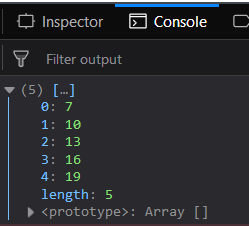4. Passing the stop less than the start parameter to the _.range() function:
Even if we pass the start parameter less than the stop parameter, the _.range() function will not give any error. It will itself adjust the step parameter as negative to reach the stop from the start given. So, the list will contain numbers from 21 till 16 as the end, 15, is not included in the list.

 `` `<``html``> ` `  `  `<``head``> ` `    ``<``script` `src` `=  ` `"https://cdnjs.cloudflare.com/ajax/libs/underscore.js/1.9.1/underscore-min.js"` `> ` `    `` ` ` ` `  `  `<``body``> ` `    ``<``script` `type``=``"text/javascript"``> ` `        ``console.log(_.range(21, 15)); ` `    `` ` ` ` `  `  ` `

Output: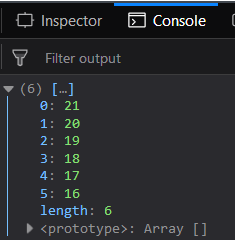NOTE:
These commands will not work in Google console or in firefox as for these additional files need to be added which they didn’t have added.

 `` `<``script` `type``=``"text/javascript"`  `src` `=``"https://cdnjs.cloudflare.com/ajax/libs/underscore.js/1.9.1/underscore-min.js"``> ` ` `

An example is shown below: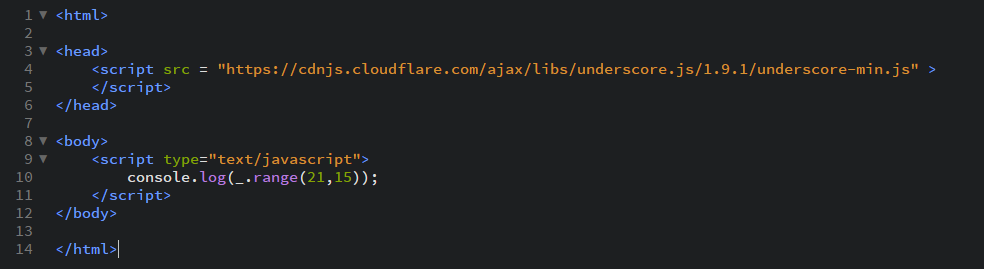My Personal Notes arrow_drop_upI like to do coding in C++C and java programming languages HTML and CSS always intersts me Sharing knowleged is the best way according to me to increase ones knwoledge

If you like GeeksforGeeks and would like to contribute, you can also write an article using contribute.geeksforgeeks.org or mail your article to contribute@geeksforgeeks.org. See your article appearing on the GeeksforGeeks main page and help other Geeks.

Please Improve this article if you find anything incorrect by clicking on the "Improve Article" button below.

Improved By : shubham_singh

Article Tags :

Be the First to upvote.

Please write to us at contribute@geeksforgeeks.org to report any issue with the above content.